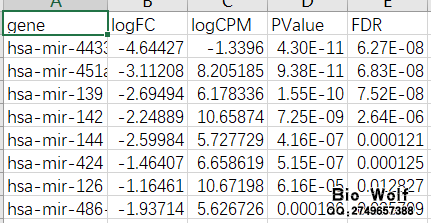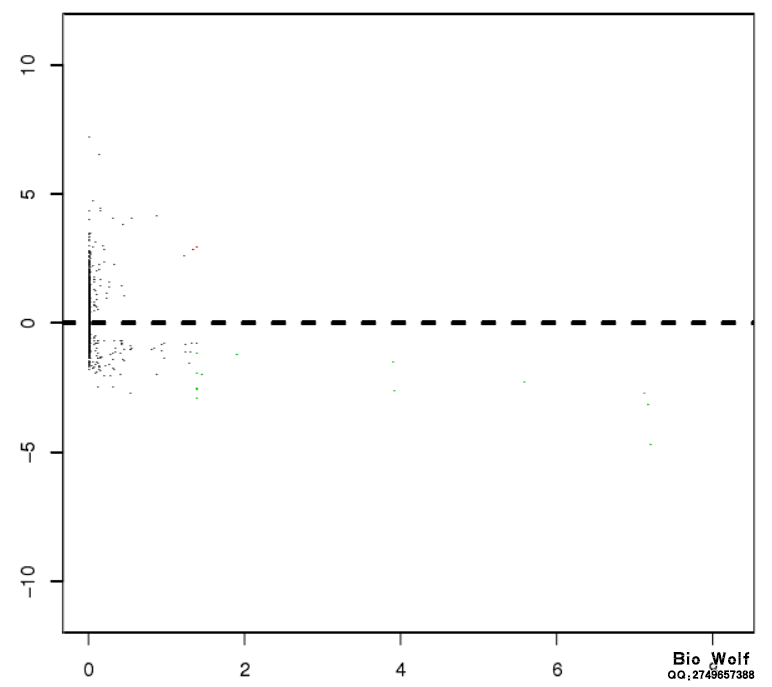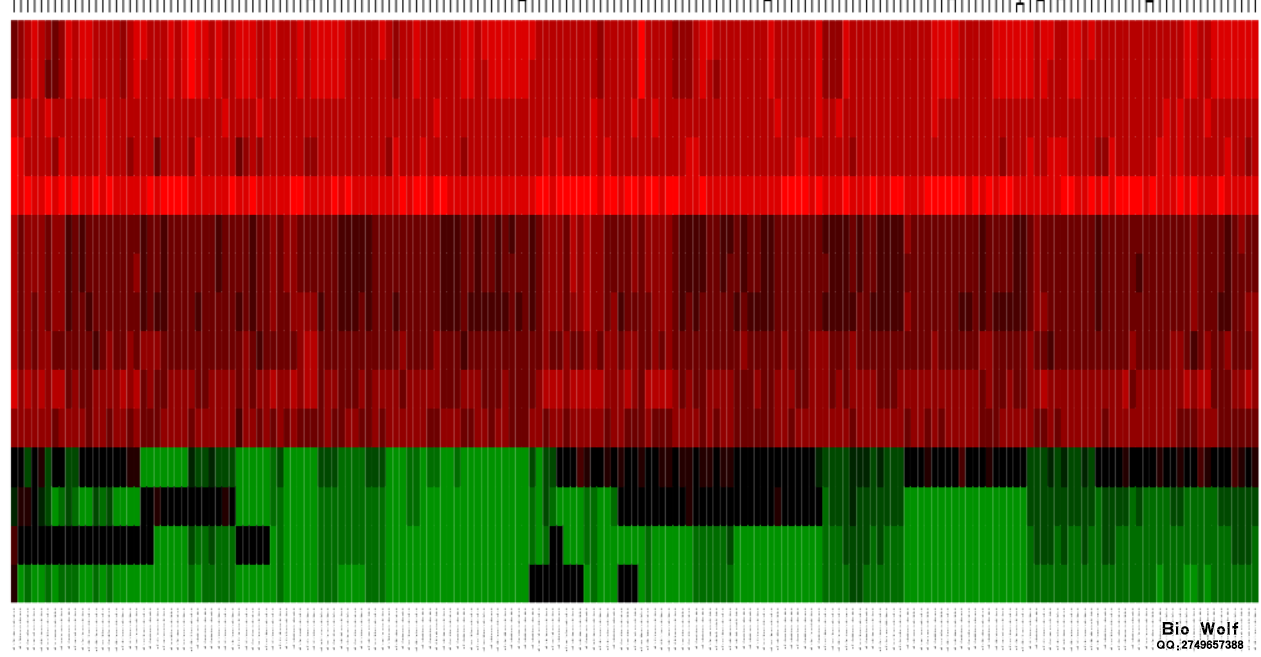### TCGAmiRNA做差异表达分析

TCGA数据库挖掘涉及很多内容，这篇推文给大家讲解的是如何做TCGA数据miRNA差异表达分析

 ```source("http://bioconductor.org/biocLite.R") biocLite("edgeR") install.packages("gplots")```

 ```foldChange=1 padj=0.05 setwd("目录") #设置工作目录 library("edgeR") bio=read.table("biowolf.txt",sep="\t",header=T,check.names=F) #改成自己的文件名 bio=as.matrix(bio) rownames(bio)=bio[,1] biowolf=bio[,2:ncol(bio)] dimnames=list(rownames(biowolf),colnames(biowolf)) bw=matrix(as.numeric(as.matrix(biowolf)),nrow=nrow(biowolf),dimnames=dimnames) bw=avereps(data) bw=bw[rowMeans(bw)>0,] ... ...```BioWolf二维码生成器

(3)
50%

(3)
50%
------分隔线----------------------------